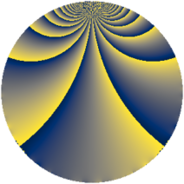# Properties

 Label 648.1.rLevel $648$ Weight $1$ Character orbit 648.r Rep. character $\chi_{648}(19,\cdot)$ Character field $\Q(\zeta_{18})$ Dimension $6$ Newform subspaces $1$ Sturm bound $108$ Trace bound $0$

# Learn more

## Defining parameters

 Level: $$N$$ $$=$$ $$648 = 2^{3} \cdot 3^{4}$$ Weight: $$k$$ $$=$$ $$1$$ Character orbit: $$[\chi]$$ $$=$$ 648.r (of order $$18$$ and degree $$6$$) Character conductor: $$\operatorname{cond}(\chi)$$ $$=$$ $$216$$ Character field: $$\Q(\zeta_{18})$$ Newform subspaces: $$1$$ Sturm bound: $$108$$ Trace bound: $$0$$

## Dimensions

The following table gives the dimensions of various subspaces of $$M_{1}(648, [\chi])$$.

Total New Old
Modular forms 54 18 36
Cusp forms 18 6 12
Eisenstein series 36 12 24

The following table gives the dimensions of subspaces with specified projective image type.

$$D_n$$ $$A_4$$ $$S_4$$ $$A_5$$
Dimension 6 0 0 0

## Trace form

 $$6q + 3q^{8} + O(q^{10})$$ $$6q + 3q^{8} + 3q^{11} - 3q^{22} - 3q^{34} - 6q^{38} + 3q^{41} - 3q^{43} - 6q^{59} - 3q^{64} - 3q^{67} - 6q^{68} + 6q^{76} + 3q^{86} + 6q^{88} - 3q^{89} - 3q^{97} + 3q^{98} + O(q^{100})$$

## Decomposition of $$S_{1}^{\mathrm{new}}(648, [\chi])$$ into newform subspaces

Label Dim. $$A$$ Field Image CM RM Traces $q$-expansion
$$a_2$$ $$a_3$$ $$a_5$$ $$a_7$$
648.1.r.a $$6$$ $$0.323$$ $$\Q(\zeta_{18})$$ $$D_{9}$$ $$\Q(\sqrt{-2})$$ None $$0$$ $$0$$ $$0$$ $$0$$ $$q-\zeta_{18}^{4}q^{2}+\zeta_{18}^{8}q^{4}+\zeta_{18}^{3}q^{8}+\cdots$$

## Decomposition of $$S_{1}^{\mathrm{old}}(648, [\chi])$$ into lower level spaces

$$S_{1}^{\mathrm{old}}(648, [\chi]) \cong$$ $$S_{1}^{\mathrm{new}}(216, [\chi])$$$$^{\oplus 2}$$